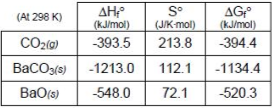# Problem: Consider the following reaction occurring at 298 K: BaCO3(s) ⇌ BaO(s) + CO2(g)What temperature is required to produce a carbon dioxide partial pressure of 1.0 atm?

###### FREE Expert SolutionStep 1: We need to determine the ΔH˚rxn:

$\overline{){\mathbf{\Delta H}}{{\mathbf{°}}}_{{\mathbf{rxn}}}{\mathbf{=}}{\mathbf{∆}}{{\mathbf{H}}}_{{\mathbf{f}}}{{\mathbf{°}}}_{{\mathbf{products}}}{\mathbf{-}}{\mathbf{∆}}{{\mathbf{H}}}_{{\mathbf{f}}}{{\mathbf{°}}}_{{\mathbf{reactants}}}}$

ΔH˚rxn = 271,500 J

Step 2: We need to determine the ΔS˚rxn:

ΔS˚rxn = 173.8 J/K

Step 3: We need to determine the ΔG˚rxn:

ΔG˚rxn = 0 J

95% (326 ratings)###### Problem Details

Consider the following reaction occurring at 298 K: BaCO3(s) ⇌ BaO(s) + CO2(g)

What temperature is required to produce a carbon dioxide partial pressure of 1.0 atm?

Frequently Asked Questions

What scientific concept do you need to know in order to solve this problem?

Our tutors have indicated that to solve this problem you will need to apply the Gibbs Free Energy concept. You can view video lessons to learn Gibbs Free Energy. Or if you need more Gibbs Free Energy practice, you can also practice Gibbs Free Energy practice problems.